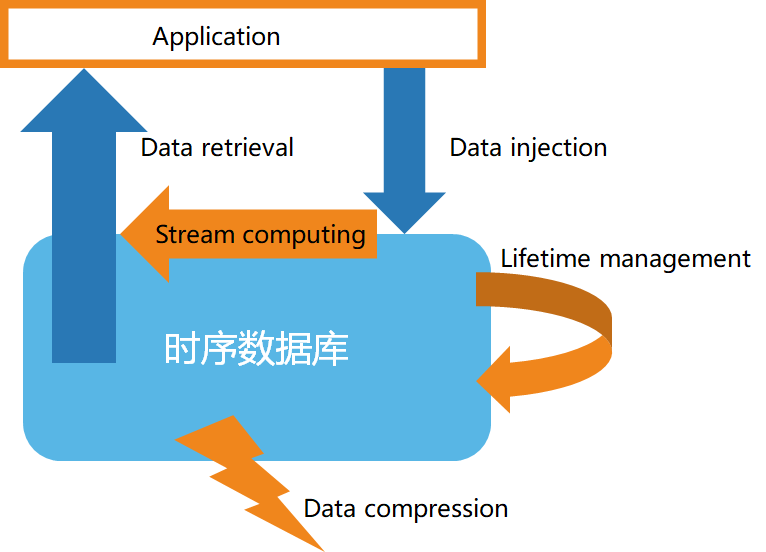# 时序数据库的流计算支持

• 历史数据的不变性
• 数据的有效性
• 数据的时效性
• 结构化的数据
• 数据的大量性• 高速的数据入库
• 数据的生命周期管理
• 数据的流处理
• 高效的数据查询
• 定制的数据压缩

1）过滤和转换 （filter & map）

2）聚合以及窗口函数 （reduce，aggregation/window）

3）多数据流合并以及模式匹配 （joining & pattern detection）

4）从流到块处理

• 案例一：使用定制化的流计算 API，如下面例子所示：

``````from(bucket: "mydb")
|> range(start: -1h)
|> filter(fn: (r) => r["_measurement"] == "mymeasurement")
|> map(fn: (r) => ({ r with value: r.value * 2 }))
|> filter(fn: (r) => r.value > 100)
|> aggregateWindow(every: 1m, fn: sum, createEmpty: false)
|> group(columns: ["location"])
|> join(tables: {stream1: {bucket: "mydb", measurement: "stream1", start: -1h}, stream2: {bucket: "mydb", measurement: "stream2", start: -1h}}, on: ["location"])
|> alert(name: "value_above_threshold", message: "Value is above threshold", crit: (r) => r.value > 100)
|> to(bucket: "mydb", measurement: "output", tagColumns: ["location"])``````

• 案例二：使用类 SQL 指令，创建流计算以及定义流计算规则，如下：

``````CREATE STREAM current_stream
TRIGGER AT_ONCE
INTO current_stream_output_stb AS
SELECT
_wstart as start,
_wend as end,
max(current) as max_current
FROM meters
WHERE voltage <= 220
INTEVAL (5S) SLIDING (1s);``````